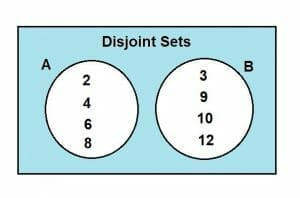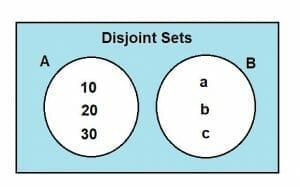WHAT

# Disjoint Sets – Explanation and Examples | Spanglers – General Blog News

45Disjoint Sets – Explanation and Examples

We have covered various sets such as finite sets, infinite sets, equal sets, null sets, universal sets, and subsets. We have also discussed the essential properties of sets in depth. But one concept remains untouched. In this article, we will be addressing this concept which is known as Disjoint Sets.

You are watching: what is pairwise disjoint

Disjoint sets are sets that do not contain any common elements. Their intersection always results in an empty or null set.

• What are disjoint sets?
• How to find if two sets are disjoint?
• Venn diagram representation of disjoint sets.
• Pairwise disjoint sets.
• Union of disjoint sets.
• Examples.
• Practice problems.

Before moving forward, you may consider refreshing your knowledge on the following prerequisites:

• Describing Sets
• Sets Notation
• Subset
• Universal Set

## What are Disjoint Sets?

As reflected from the question “what are disjoint sets?” disjoint sets are the disjoint sets. This means that such sets do not have any common elements amongst them. These sets yield a null set or an empty set upon performing the intersection’s fundamental set operation.

We can understand this statement by considering two distinct sets. Consider a set A = {a, b} and set B = {c, d}. It is quite obvious from observing these sets that they have no common element between them. The intersection of these two sets will be as follows:

A ∩ B = 𝛟

Hence, we can conclude that the intersection of disjoint sets will always result in a null set.

But is it necessary for sets to be disjoint if their intersection is a null set? To address this issue, let’s consider a group of sets. Let there be a set A = {1, 2}, a set B = {2, 3} and a set C = {3, 1}. The intersection of these three sets results in a null set, but none of these sets are disjoint sets. This is because no two sets in this group of three have a null intersection. To understand this better, let’s evaluate the intersection of these sets.

A ∩ B ∩ C = 𝛟

The joint intersection of the three sets is a null set.

The intersection of the pair of sets in the group of three sets is given as:

A ∩ B = {2}

B ∩ C = {3}

A ∩ C = {1}

No pair of sets in the three sets are disjoint sets because the pair’s intersection does not result in a null set.

To further understand the concept of disjoint sets, consider the following example.

Example 1

Consider the following sets:

A = {2, 4, 6}

B = {9, 12, 15}

Determine whether these sets are disjoint sets.

Solution

To determine if these sets are disjoint, we will perform the intersection operation on these sets.

A ∩ B = {2, 4, 6} ∩ {9, 12, 15}

A ∩ B = 𝛟

Since the intersection of sets A and B is a null set, sets A and B are disjoint sets.

## How to Find if two sets are Disjoint?

To determine whether two sets are disjoint sets, all you have to do is perform the intersection operation. Disjoint sets will never have any common element between them. Therefore, their intersection will always be a null set.

The condition for sets to be disjoint is stated below:

A ∩ B = 𝛟

If you have a group of sets instead of two sets, you need to evaluate each pair of sets’ intersection. In this case, it does not matter if the intersection of three or more sets results in a null set. To find out the disjoint sets, you will need to evaluate each pair of sets’ intersection operation in this case. Whichever pair yields a null set will contain disjoint sets.

To understand this concept, let’s solve an example.

Example 2

Consider the following group of sets:

A = {1, 2}

B = {2, 3}

C = {5, 3}

Determine which of these sets are disjoint sets.

Solution

To find out the disjoint sets, let’s first consider the intersection of these sets.

A ∩ B ∩ C = 𝛟

Now, let’s apply the intersection operation on each pair of sets.

A ∩ B = {1, 2} ∩ {2, 3}

A ∩ B = {2}

And,

B ∩ C = {2, 3} ∩ {5, 3}

B ∩ C = {3}

And finally the last pair,

A ∩ C = {1, 2} ∩ {5, 3}

A ∩ C = 𝛟

So, in this group of sets, only sets A and C are disjoint sets.

## Venn Diagram Representation of Disjoint Sets

A Venn diagram is the most accurate and comprehensive tool used to depict the relationship between two or more sets. We can portray all of the essential set operations by using a Venn diagram.

We can use Venn diagrams to indicate disjoint sets. We already mentioned above that disjoint sets do not have any common element and have a null intersection. Adhering to this statement, the Venn diagram of disjoint sets has no overlapping region between the two sets.

To understand the Venn diagram depiction of disjoint sets, consider two sets, A and B. Let A = {2, 4, 6, 8} and let B = {3, 9, 12, 10}. The Venn diagram of these two sets is shown below:Since the two sets, A and B, do not have any intersecting element, these are disjoint sets, and their Venn diagram will not have any overlapping region.

Let’s solve an example to understand this concept further.

Example 3

Consider two sets:

A = {10, 20, 30}

B = {a, b, c}

Show their relationship through a Venn diagram.

Solution

It is evident by analyzing the two sets that they do not have any intersecting elements, hence,

A ∩ B = 𝛟

So, sets A and B are disjoint sets.

The Venn diagram representation of these sets is shown below:## Pairwise Disjoint Sets

Now that we have developed an understanding of disjoint sets, let’s address the concept that usually tangles up young mathematics enthusiasts’ minds. Often they encounter the term ‘Pairwise’ while dealing with disjoint sets. This term should not create any misconception because pairwise disjoint sets have the same disjoint sets discussed above.

Pairwise disjoint sets are the pair of sets whose intersection is a null set. Such sets do not have any common element between them. Therefore, pairwise disjoint sets are the same as disjoint sets. The criteria for two sets to be pairwise disjoint sets is given below:

A ∩ B = 𝛟

If we have a set of sets, then any pair of sets in that group that yields a null intersection will be declared as pairwise disjoint sets.

Let’s solve an example regarding the pairwise disjoint sets.

Example 4

Consider the following two sets:

A = {5, 7, 9}

B = {1, 2, 3}

Determine if these two sets are pairwise disjoint sets.

Solution

The criteria for pairwise disjoint sets is given as:

A ∩ B = 𝛟

Performing the intersection operation on sets A and B:

A ∩ B = {5, 7, 9} ∩ {1, 2, 3}

A ∩ B = 𝛟

Therefore, the two sets A and B are pairwise disjoint sets.

## Union of Disjoint Sets

We have already discussed the fundamental set operation of the union in our previous lessons. But to given a quick recap, the union of any two or more sets contains all the elements enveloped individually in each set.

The union of disjoint sets is slightly different from the conventional union of sets. The disjoint union is a binary operation performed on two disjoint sets. Before performing the disjoint union operation, the sets must maintain their disjoint status even after performing the union operation. For this purpose, we must alter each disjoint set before getting enveloped in a disjoint union. Emphasizing this statement, the disjoint union needs to follow the following convention:

A U* B = { A x (0) } U { B x (1) }

Where U* is the disjoint union and sets A and B are disjoint sets. The disjoint union is a bijective operation.

This convention helps obtain the disjoint sets’ union and ensures that the disjoint sets retain their disjoint identity.

Let’s solve some examples to understand the concept of disjoint union.

Example 5

Consider the two disjoint sets:

A = {a, b, c}

B = {d, e, f}

Find the union of these sets.

Solution

Since sets A and B are disjoint sets, hence their union will be a disjoint union operation.

So, the disjoint union is:

A U* B = {(a,0), (b,0), (c,0)} U {(d, 1), (e, 1), (f, 1)}

So,

A U* B = { (a,0), (b,0), (c,0), (d,1), (e,1), (f,1) }

Where A U* B is the disjoint union of the disjoint sets A and B.

Example 6

Consider the following sets:

A = {2, 3, 4}

B = {5, 6, 7}

Find out the union of these sets.

Solution

Before finding the union of these two sets, first, let’s find out if they are disjoint sets or not.

The condition for disjoint sets is:

A ∩ B = 𝛟

Finding the intersection,

A ∩ B = {2, 3, 4} ∩ {5, 6, 7}

A ∩ B = 𝛟

As the intersection of sets A and B is a null set, hence these sets are disjoint.

Finding the union,

A U* B = {(2,0), (3,0), (4,0)} U {(5,1), (6,1), (7,1)}

Hence,

A U* B = {(2,0), (3,0), (4,0), (5,1), (6,1), (7,1)}

This union is the disjoint union.

To further strengthen the concept of disjoint sets, consider the following practice problems.

### Practice Problems

1. Determine if the sets A = {34, 55, 78} and B = {2, 3, 4} are disjoint sets.
2. Determine if the sets A = {set of 5 working days} and B = {weekends} are disjoint sets.
3. Find the pairwise disjoint sets in the group of sets X = {2, 4, 6}, Y = {3, 6, 9} and Z = {6, 9}.
4. Find the union of the sets {vowels} and {constants}.
5. Find the union of the sets {8, 9} and {10, 11}.

1. Disjoint sets
2. Disjoint sets
3. No pairwise disjoint sets
4. {(vowels, 0), (constants, 1)}
5. {(8,0), (9,0), (10,1), (11,1)}